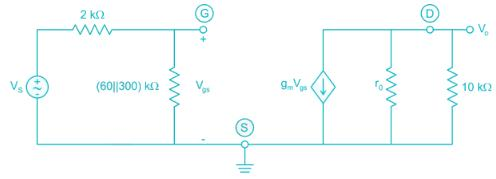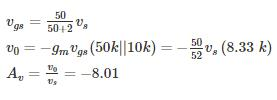Courses

# Analog Electronics - 3

## 10 Questions MCQ Test RRB JE for Electronics & Communication Engineering | Analog Electronics - 3

Description
This mock test of Analog Electronics - 3 for Electronics and Communication Engineering (ECE) helps you for every Electronics and Communication Engineering (ECE) entrance exam. This contains 10 Multiple Choice Questions for Electronics and Communication Engineering (ECE) Analog Electronics - 3 (mcq) to study with solutions a complete question bank. The solved questions answers in this Analog Electronics - 3 quiz give you a good mix of easy questions and tough questions. Electronics and Communication Engineering (ECE) students definitely take this Analog Electronics - 3 exercise for a better result in the exam. You can find other Analog Electronics - 3 extra questions, long questions & short questions for Electronics and Communication Engineering (ECE) on EduRev as well by searching above.
QUESTION: 1

### When the gate-to-source voltage VGS of a MOSFET with a threshold voltage of 400mV, working in saturation is 900mV, the drain current is observed to be 1mA. Neglecting the channel width modulation effect and assuming that the MOSFET is operating at saturation, the drain current for an applied VGS of 1400mV is

Solution:

The MOSFET current is saturation is given by,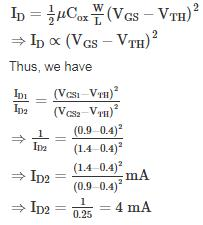*Answer can only contain numeric values
QUESTION: 2

### What percentage of current IDSS is the drain current for a JFET, if the gate to source voltage is 65% of the pinch-off voltage.

Solution:

VGS = 0.65 Vp

The drain current of JFET is given as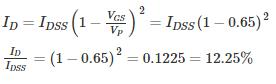QUESTION: 3

### The ‘Pinch-off’ voltage of a JFET is 4 V. Then the off gate voltage of JFET is

Solution:

The Cut off gate voltage is also known as pinch off voltage at which the drain current becomes zero.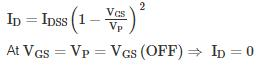*Answer can only contain numeric values
QUESTION: 4

A MOSFET operating in saturation mode is shown in the below figure. The drain current is given as ID = ½ (VGS – 0.75)2 mA If VS = 5V, then the value of RS­­­ in kΩ is __________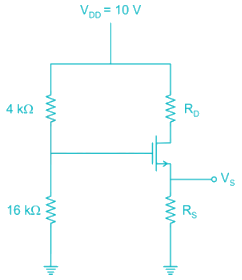Solution:

The bias used in the given circuit is voltage divider bias. The gate voltage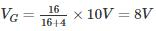∴ Gate source voltage VGS = VG - VS

= 8 – 5

= 3 V

∴ ID = ½ (3 - 0.75)2 mA

≅ 2.53 mA

VS = RSID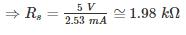*Answer can only contain numeric values
QUESTION: 5

For the amplifier shown below, the drain current changes from 5 mA to 7 mA when the gate voltage D changed from -4.0 V to -3.7 V. The voltage gain of the amplifier is _____

Solution:

The gain of the amplifier is given by:

Av = - gmrD

gm: trans conductance of transistor

rD: effective AC resistance seen by drain terminal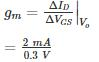gm = 6.66 ms

rD = RD||RL = 6k||6k = 3 kΩ

Av = -6.66 × 10-3 × 3 × 103

Av = -19.98 ≈ 20

QUESTION: 6

The NMOS transistors in the circuit below have VT = 1 V, μncox = 120 μA/V2, λ = 0 and L1 = L2 = L3 = 1 μm. The values of width of each of Q1, Q2, Q3 such that the voltage values are as shown in below figure are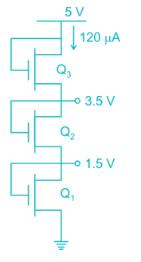Solution:

Let ID be the drain current, it will be common for all three transistors. All the transistors are operating in saturation since VD = VG

At transistor Q3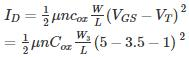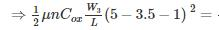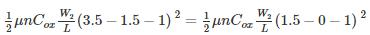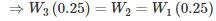Given ID = 120 μA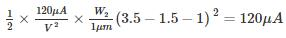⇒ W2 = 2 μm

*Answer can only contain numeric values
QUESTION: 7

A common source amplifier biased at ID = 0.25 mA with RD = 20 kΩ and early voltage VA = 50 V and overdrive voltage (VGS – VT)DC = 0.25 V. If the input is fed through 100 kΩ and output are taken from 20 kΩ loads then the overall gain of the amplifier is _____ V/V.

Solution:

The Ac equivalent circuit can be drawn as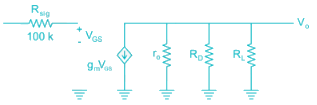ID = 0.25 mA; VA = 50 V; Vov (= VGs - VT) = 0.25 V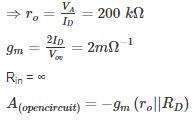(∵ rO >> RD)

= -36.36 V/V

Av(Overall gain) = -gm(R|| r|| RL)

= -2 mΩ-1 × 9.53 kΩ

≃ -19 V/V

QUESTION: 8

The transistor I the circuit is shown below has parameters IDSS = 8 mA and VP = -4 V. The value of VDS is___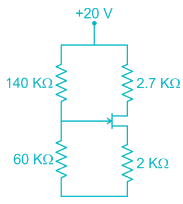Solution: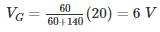Assume the transistor in saturation,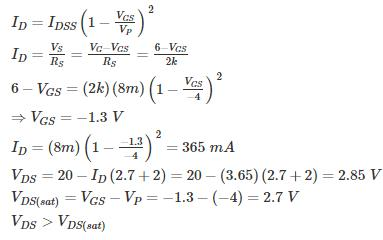Assumption is correct.

Hence VDS = 2.85 V.

*Answer can only contain numeric values
QUESTION: 9

The small signal resistance (dVC/dID) of the n-channel MOSFET shown in figure below at bias voltage VC = 2V is (in kΩ) _________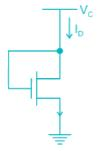Given μnCox W/L = 40μ A/V2

VT = 1 V

Solution:

Since VDS = VGS the device is in saturation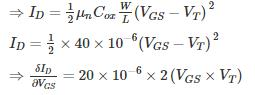= 40 × 10-6 (2 - 1)

Hence small signal resistance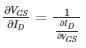= 25 kΩ

*Answer can only contain numeric values
QUESTION: 10

In the circuit shown below the parameters are gm =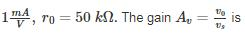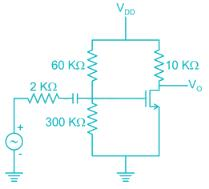Solution:

The small signal equivalent circuit is as shown below